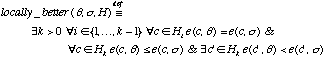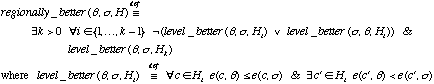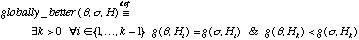Guide to Constraint Programming © Roman Barták, 1998 Contents Prev Up Next

# Constraint Hierarchies

[definitions] [comparators] [solutions] [inter-hierarchy] [non-monotony]

Constraint hierarchies were introduced for describing over-constrained systems of constraints by specifying constraints with hierarchical strengths or preferences. It allows one to specify declaratively not only the constraints that are required to hold, but also weaker, so called soft constraints at an arbitrary but finite number of strengths. Weakening the strength of constraints helps to find a solution of previously over-constrained system of constraints. Intuitively, the hierarchy does not permit to the weakest constraints to influence the result. Moreover, constraint hierarchies allow "relaxing" of constraints with the same strength by applying, e.g., weighted-sum, least-squares or similar comparators.

### Example in Constraint Hierarchies

Consider the problem of choosing matching clothes from the introductory section to over-constrained problems. Now, we can specify labeled constraints which express our preferences about matching clothes.
S::{r,w}, F::{c,s}, T::{b,d,g}
```required CST::{(r,g),(w,b),(w,d)}
strong CFT::{(s,d),(c,g)}
weak CSF::{(w,c)}.```

Using any comparator (see thereinafter) will give two solutions: (S,F,T)=(r,c,g) and (S,F,T)=(w,s,d), that satisfy the required and strong constraints but violates the weak constraint. Note, that there is no valuation satisfying all the constraints and that the above two valuations satisfy the priority constraints and, thus, they comprise the solution.

###Formal definitions

In constraint hierarchies, one uses labeled constraints which are constraints labeled with a strength or preference. Then, a constraint hierarchy is a (finite) multiset of labeled constraints. Given a constraint hierarchy H, let H0 denote the required constraints in H, with their labels removed. In the same way, we define the sets H1, H2, ... for levels 1, 2, ...

A solution to a constraint hierarchy H will consist of valuations for variables in H, that satisfy best constraints in H respecting the hierarchy. Formally (U, V are valuations):

SH,0 = {U | for each c in H0 , c is satisfied by U}
SH = {U | U is from SH,0 & for each V in SH,0 V is not better than U respecting H}.

The set SH,0 contains valuations satisfying required constraints in H and SH, called a solution set for H, is a subset of SH,0 containing all valuations which are not worse than other valuations in SH,0.

###Comparators

There is a number of reasonable candidates for the predicate better, in the above definition of solution set, that is called a comparator. A comparator is an irreflexive and transitive relation over valuations of variables that respects the hierarchy, i.e., if there is some valuation in S0 that completely satisfies all the constraints through level k, then all valuations in S must satisfy all the constraints through level k. Note that the comparator defines partial order over valuations.

Currently, there are three widely used groups of comparators (respective valuations are denoted by greek symbols in formal definitions):

locally-better comparators

consider each constraint individually, i.e., a valuation U is locally-better than another valuation V if, for each of the constraints through some level k-1, the errors are equal for respective valuations, and at level k the error is strictly less for at least one constraint and less than or equal for all the restregionally-better comparators

extend locally-better comparators to enable comparison of valuations which are incomparable by locally-better comparators, i.e., a valuation U is regionally-better than another valuation V if, for each level till k-1, the levels are incomparable by locally-better comparator, and at level k the error is strictly less for at least one constraint and less than or equal for all the rest.globally-better comparators

consider combined errors of individual constraints at each level, i.e., a valuation U is globally-better than another valuation V if, the combined errors are equal till some level k-1 for both valuations, and at level k the combined error is strictly less for the valuation U.There is a number of reasonable candidates for the combining function that combines error of individual constraints at each level, namely weighted-sum, worst-case or least-squares methods.

The above defined comparators need an error function e(c,V) that returns a nonnegative real number indicating how nearly the constraint c is satisfied for a valuation V. The trivial error function returns 0 if the constraint is satisfied and 1 otherwise. Comparators based on this error function are called predicate-comparators. If some metric is used as the error function that the respective comparator is called metric-comparator.

###Existence of Solutions

In the above section we give a formal definition of the solution of the hierarchy. Now, the question is:
"If the set of solutions SH,0 for the required constraints is non-empty, is the set SH of solutions for the hierarchy non-empty as well?"

Intuitively one might expect the answer yes, but there are some pathological hierarchies for which this is not the case. The following example presents such hierarchy.

 Example: Consider the hierarchy {required X>0, prefer X=0} for the domain of the real numbers, using a metric comparator. Then SH,0 consist of all valuations mapping X to a positive number. Let X=d be such valuation. Then, we can find another valuation, for example X=d/2, that better satisfies the soft constraint X=0. Therefore, the set SH of solutions for the hierarchy is empty because for every valuation in SH,0 it is possible to find a better valuation in SH,0.

By analyzing the above example it is possible to identify three sources of this pathological behaviour and to formulate the following propositions that guarantee the existence of the solution under some conditions.

 Proposition 1: If the set SH,0 is non-empty and finite then the set SH is non-empty. Proof: Suppose to the contrary that SH is empty. Pick any valuation V1 from SH,0 (SH,0 is non-empty). According to the assumption that SH is empty, V1 does not belong to SH. and therefore there is an V2 in SH,0 such that V2 is better than V1 respecting H. Similarly , since V2 does not belong to SH, there is an V3 in SH,0 such that V3 is better than V2, and so forth for an infinite chain V4, V5, ... Since the comparator better is irreflexive and transitive, it follows that all the Vi are distinct, and so there are an infinite number of them. But, by hypothesis SH,0 is finite, a contradiction.

 Proposition 2: If the set SH,0 is non-empty and if a predicate comparator is used, then the set SH is non-empty. Proof: Suppose to the contrary that SH is empty. Using the same argument as before, we show that there must be an infinite number of distinct valuations Vi in SH,0. However, if the comparator is predicate, one valuation cannot be better than another valuation if both valuations satisfy exactly the same subset of constraints in H. Therefore each of the Vi must satisfy a different subset of the constraints in H. However, this is a contradiction, since H is finite.

 Proposition 3: If the set SH,0 is non-empty and D is a finite domain then the set SH is non-empty. Proof: Because the hierarchy is a finite set, it contains only a finite number of variables. The domain D of variables is also finite and, therefore, there exists only finite number of valuations. Consequently, the set SH,0 is finite. Now, both conditions of Proposition 1 are satisfied and therefore we can derive that the set SH is non-empty.

###Inter-Hierarchy Comparison

In the original definition of constraint hierarchies, only alternate solutions to one constraint hierarchy are compared. The later extension also supports comparison of solutions to more constraint hierarchies, so called inter-hierarchy comparison (opposite to traditional intra-hierarchy comparison described above).

Inter-hierarchy comparison uses globally-better comparators to compare valuations arising from more hierarchies (neither locally-better nor regionally better comparators can do it). Formally (X is a set of constraint hierarchies):

SX,0 = {UH | H is in X & for each c in H0 , c is satisfied by UH}
SX = {UH | UH is from SX,0 & for each VJ in SX,0, VJ is not better than UH respecting X}.

Note that each valuation is labeled by it's respective hierarchy now.

Inter-hierarchy comparison is useful especially in Hierarchical Constraint Logic Programming (HCLP), where it can rule out some unintuitive solutions. HCLP is an extension of CLP (Constraint Logic Programming) that uses constraint hierarchies instead of flat constraints.

###Nonmonotonic Aspects

Classical logic is monotonic in the sense that adding new axioms to a theory can only enlarge the set of statements that can be inferred. It is never necessary to retract conclusions as new knowledge is gained. If the inference system is monotony then it is possible to infer new solution after adding new axioms directly from the original solution. Unfortunately such monotony feature is not present in constraint hierarchies and, thus, the constraint hierarchy solvers must (in general) solve the new hierarchy after adding new constraints from scratch.

We say that the comparator is orderly, if the solution set does not enlarge after adding new constraints, i.e., SH union J is subset of SH , where H and J are constraint hierarchies. A comparator that is not orderly is disorderly.

Unfortunately, any comparator that respects the hierarchy is disorderly. In general, this feature prevents construction of incremental constraint hierarchy solvers.

 Proposition: Let D be a nontrivial domain (i.e., a domain with more than one element). Then any comparator that respects the hierarchy is disorderly. Proof: Let H={weak X=a} and let J={strong X=b}, where a and b are two distinct elements in D. Then the solution of the hierarchy H is X=a using arbitrary comparator C that respects the hierarchy, while the solution of the hierarchy (H union J) is X=b that is not a subset of the former solution. Therefore the comparator C is not orderly.

It is also possible to define similar feature of inter-hierarchy comparison.

We say that the comparator is monotonic if the solution set of the set of hierarchies does not reduce after adding new constraint hierarchies to this set, i.e., SX is subset of SX union Y , where X and Y are sets of constraint hierarchies. A comparator that is not monotonic is nonmonotonic.

Again, any comparator respecting the set of hierarchies is nonmonotonic.

 Proposition: Let D be a nontrivial domain. Then any comparator that respects the set of hierarchies is nonmonotonic. Proof: Let X={{required A=a, weak A=b}} and Y={{required A=b}}, where a and b are two distinct elements in D. Then the solution of the set of hierarchies X (in fact, X contains exactly one hierarchy) is A=a using arbitrary comparator C that respects the set of hierarchies, while the solution of the set of hierarchies (X union Y) (this set contains two hierarchies) is A=b because this solution satisfies all constraints of the respective hierarchy {required A=b}. This is not a superset of the former solution and therefore the comparator C is not monotonic.

[definitions] [comparators] [solutions] [inter-hierarchy] [non-monotony]

 Contents Prev Up Next Designed and maintained by Roman Barták